# Newton’s Laws of MotionPage 3

#### WATCH ALL SLIDES

1. What acceleration will result when a 12 N net force applied to a 3 kg object?

12 N = 3 kg x 4 m/s/s

2. A net force of 16 N causes a mass to accelerate at a rate of 5 m/s2. Determine the mass.

16 N = 3.2 kg x 5 m/s/s

3. How much force is needed to accelerate a 66 kg skier 1 m/sec/sec?

66 kg-m/sec/sec or 66 N

4. What is the force on a 1000 kg elevator that is falling freely at 9.8 m/sec/sec?

9800 kg-m/sec/sec or 9800 N

Slide 22Slide 23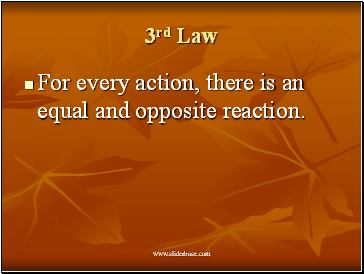3rd Law

For every action, there is an equal and opposite reaction.

Slide 24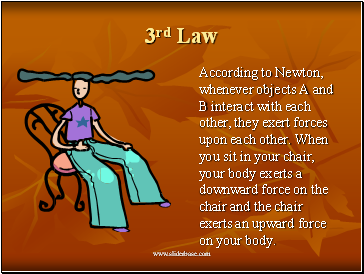3rd Law

According to Newton, whenever objects A and B interact with each other, they exert forces upon each other. When you sit in your chair, your body exerts a downward force on the chair and the chair exerts an upward force on your body.

Slide 253rd Law

There are two forces resulting from this interaction - a force on the chair and a force on your body. These two forces are called action and reaction forces.

Slide 26## Newton’s 3rd Law in Nature

Consider the propulsion of a fish through the water. A fish uses its fins to push water backwards. In turn, the water reacts by pushing the fish forwards, propelling the fish through the water.

The size of the force on the water equals the size of the force on the fish; the direction of the force on the water (backwards) is opposite the direction of the force on the fish (forwards).

Slide 27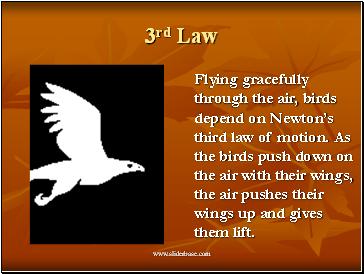3rd Law

Flying gracefully through the air, birds depend on Newton’s third law of motion. As the birds push down on the air with their wings, the air pushes their wings up and gives them lift.

Slide 28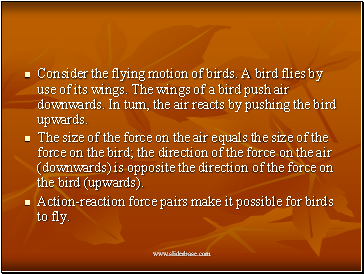Consider the flying motion of birds. A bird flies by use of its wings. The wings of a bird push air downwards. In turn, the air reacts by pushing the bird upwards.

The size of the force on the air equals the size of the force on the bird; the direction of the force on the air (downwards) is opposite the direction of the force on the bird (upwards).

Action-reaction force pairs make it possible for birds to fly.

Slide 29Slide 30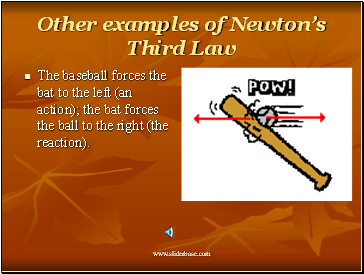Other examples of Newton’s Third Law

Go to page:
1  2  3  4